# 代码详解：卷积神经网络介绍

+关注继续查看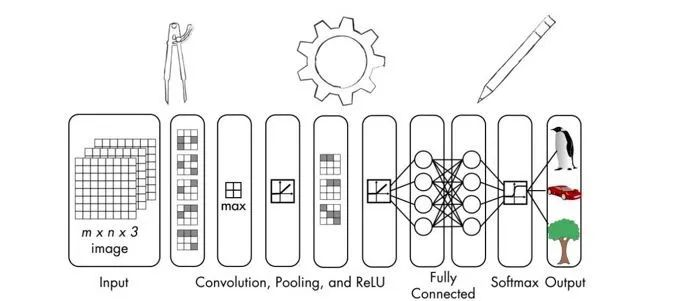CNN也是由具有权重和偏差的神经元组成。这些神经元接收输入的数据并处理，然后输出信息。神经网络的目标是将输入层中的原始图像数据转到输出层中的正确类中。普通神经网络和CNN之间的区别在于使用的层类型以及处理输入数据的方式。假设CNN的输入是图像，这允许其提取特定于图像的属性。这使得CNN在处理图像方面更有效率。那么，CNN是如何构建的？

CNN的体系结构

CNN在处理数据时明确考虑图像的结构。CNN中的神经元按三维排列——宽度、高度和深度。当前层中的每个神经元都连接到前一层输出的小块。这就像在输入图像上叠加NxN过滤器一样。这与完全连接的层相反，完全连接层的每个神经元均与前一层的所有神经元相连。

CNN中的层类型

· 输入层：用于原始图像数据的输入。

· 卷积层：该层计算神经元与输入中各种切片之间的卷积。

· 激励层：此图层将激活函数应用于前一图层的输出。该函数类似于max（0，x）。需要向该层神经网络增加非线性映射，以便它可以很好地概括为任何类型的功能。

· 池化层：此层对前一层的输出进行采样，从而生成具有较小维度的结构。在网络中处理图像时，池化有助于只保留突出的部分。最大池是池化层最常用的，可在给定的KxK窗口中选择最大值。

· 全连接层：此图层计算最后一层的输出分。输出结果的大小为1x1xL，其中L是训练数据集中的类数。

https：//www.tensorflow.org/get_started/os_setup。

import numpy as np

import matplotlib.pyplot as plt

import tensorflow as tf

# Define the number of points to generate

num_points = 1200

# Generate the data based on equation y = mx + c

data = []

m = 0.2

c = 0.5

for i in range(num_points):

# Generate 'x'

x = np.random.normal(0.0, 0.8)

# Generate some noise

noise = np.random.normal(0.0, 0.04)

# Compute 'y'

y = m*x + c + noise

data.append([x, y])

# Separate x and y

x_data = [d for d in data]

y_data = [d for d in data

# Plot the generated data

plt.plot(x_data, y_data, 'ro')

plt.title('Input data')

plt.show()

# Generate weights and biases

W = tf.Variable(tf.random_uniform(, -1.0, 1.0))

b = tf.Variable(tf.zeros())

# Define equation for 'y'

y = W * x_data + b

# Define how to compute the loss

loss = tf.reduce_mean(tf.square(y - y_data))

# Define the gradient descent optimizer

train = optimizer.minimize(loss)

# Initialize all the variables

init = tf.initialize_all_variables()

# Start the tensorflow session and run it

sess = tf.Session()

sess.run(init)

# Start iterating

num_iterations = 10

for step in range(num_iterations):

sess.run(train)

# Print the progress

print('nITERATION', step+1)

print('W =', sess.run(W))

print('b =', sess.run(b))

print('loss =', sess.run(loss))

# Plot the input data

plt.plot(x_data, y_data, 'ro')

# Plot the predicted output line

plt.plot(x_data, sess.run(W) * x_data + sess.run(b))

# Set plotting parameters

plt.xlabel('Dimension 0')

plt.ylabel('Dimension 1')

plt.title('Iteration ' + str(step+1) + ' of ' + str(num_iterations))

plt.show()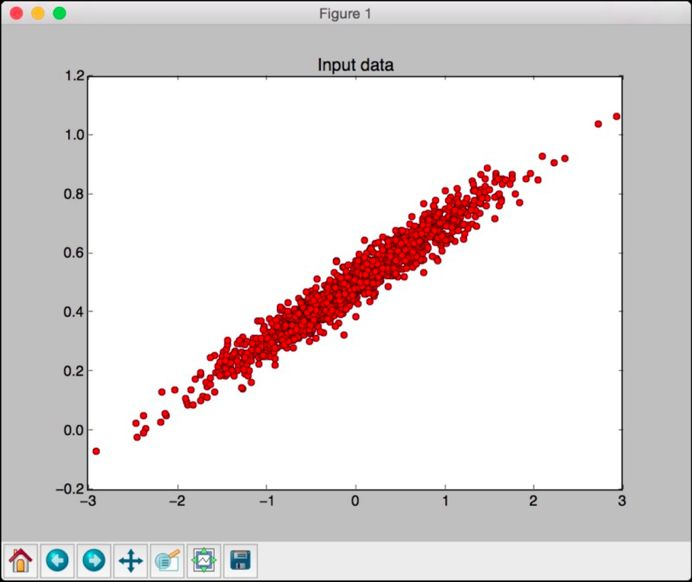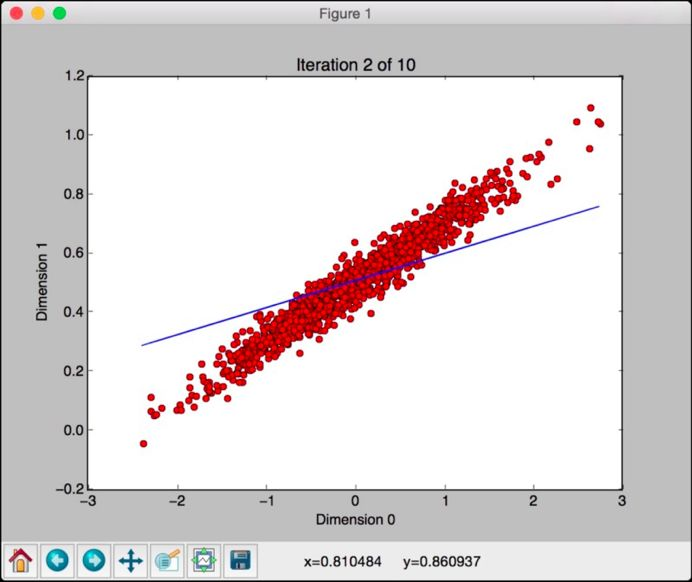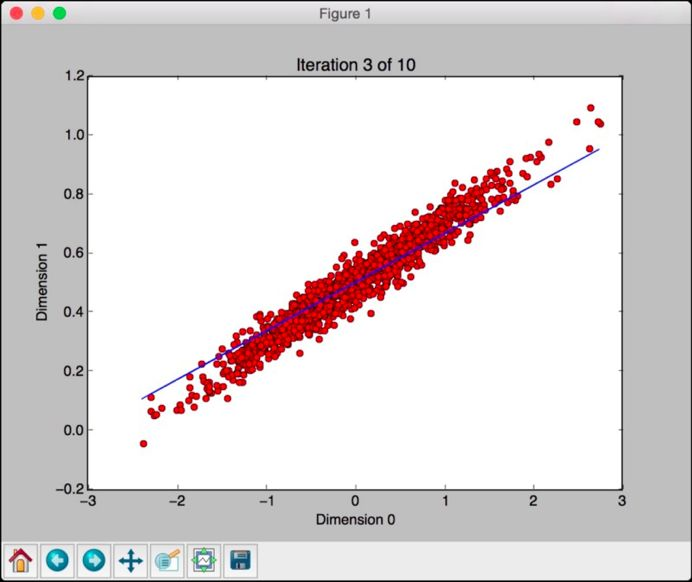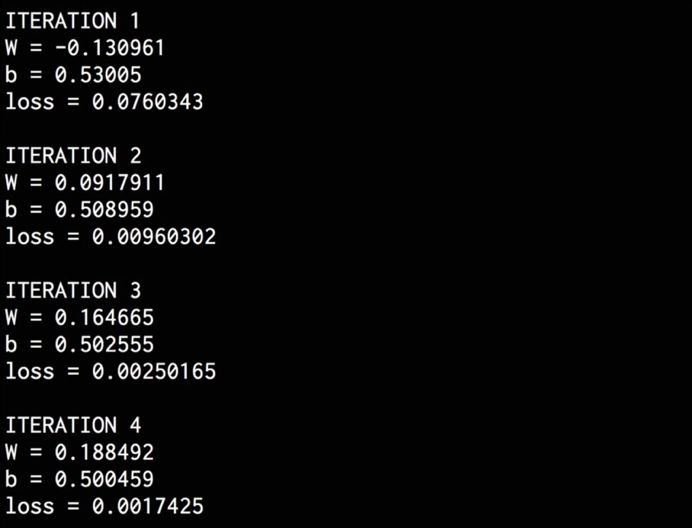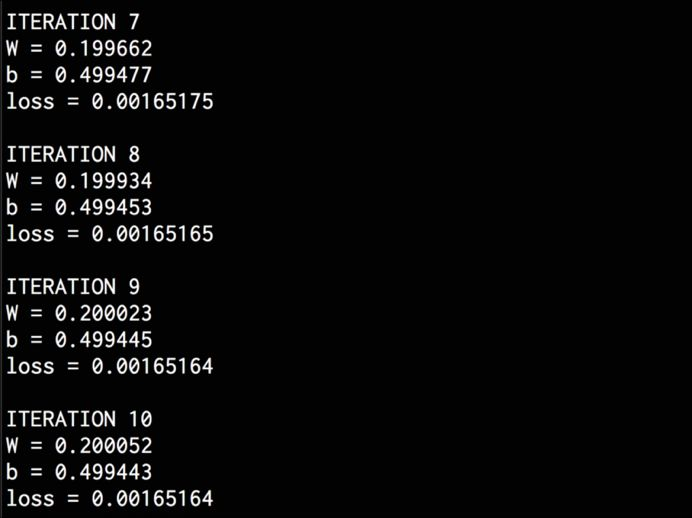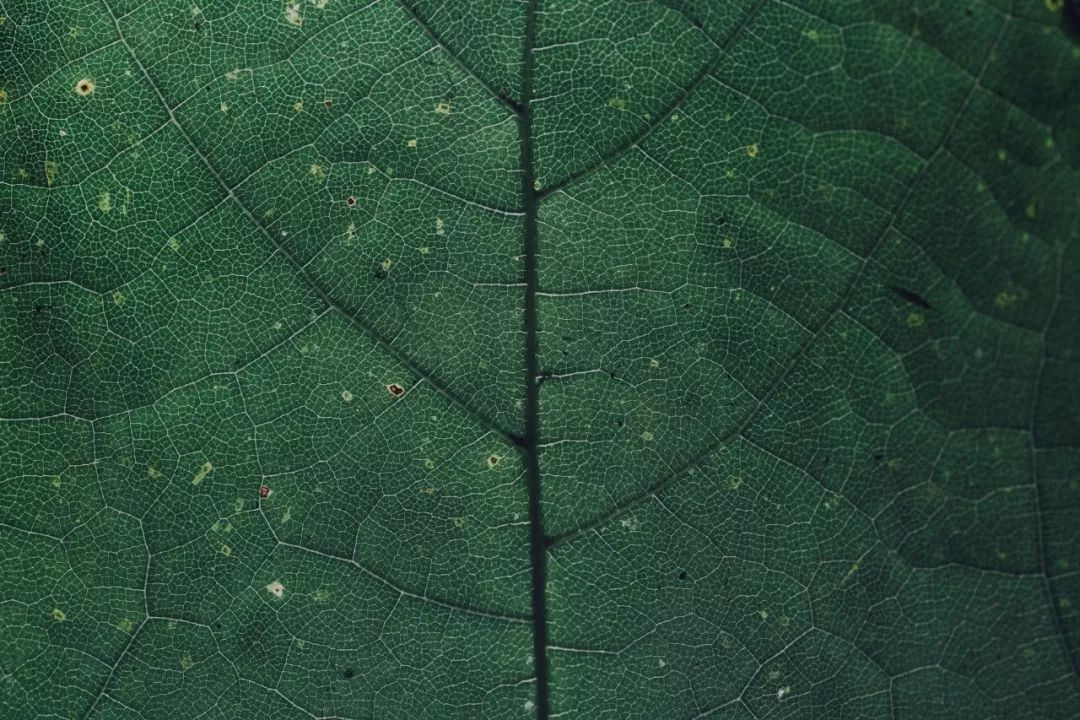import argparse

import tensorflow as tf

from tensorflow.examples.tutorials.mnist import input_data

def build_arg_parser():

parser = argparse.ArgumentParser(description='Build a classifier using

MNIST data')

default='./mnist_data', help='Directory for storing data')

return parser

if name == '__main__':

args = build_arg_parser().parse_args()

# with 784 neurons (28x28=784)

x = tf.placeholder(tf.float32, [None, 784])

# digits, so the output layer should have 10 classes

W = tf.Variable(tf.zeros([784, 10]))

b = tf.Variable(tf.zeros())

# Create the equation for 'y' using y = W*x + b

y = tf.matmul(x, W) + b

# Define the entropy loss and the gradient descent optimizer

y_loss = tf.placeholder(tf.float32, [None, 10])

loss = tf.reduce_mean(tf.nn.softmax_cross_entropy_with_logits(y, y_loss))

# Initialize all the variables

init = tf.initialize_all_variables()

# Create a session

session = tf.Session()

session.run(init)

# Start training

num_iterations = 1200

batch_size = 90

for _ in range(num_iterations):

# Get the next batch of images

x_batch, y_batch = mnist.train.next_batch(batch_size)

# Train on this batch of images

session.run(optimizer, feed_dict = {x: x_batch, y_loss: y_batch})

# Compute the accuracy using test data

predicted = tf.equal(tf.argmax(y, 1), tf.argmax(y_loss, 1))

accuracy = tf.reduce_mean(tf.cast(predicted, tf.float32))

print('nAccuracy =', session.run(accuracy, feed_dict = {

x: mnist.test.images,

y_loss: mnist.test.labels}))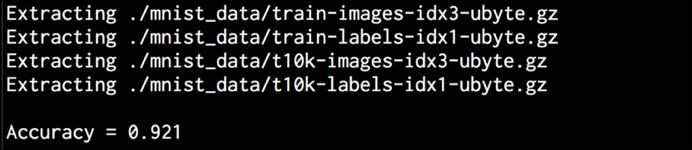import argparse

import tensorflow as tf

from tensorflow.examples.tutorials.mnist import input_data

def build_arg_parser():

parser = argparse.ArgumentParser(description='Build a CNN classifier

using MNIST data')

default='./mnist_data', help='Directory for storing data')

return parser

def get_weights(shape):

data = tf.truncated_normal(shape, stddev=0.1)

return tf.Variable(data)

def get_biases(shape):

data = tf.constant(0.1, shape=shape)

return tf.Variable(data)

def create_layer(shape):

# Get the weights and biases

W = get_weights(shape)

b = get_biases([shape[-1]])

return W, b

def convolution_2d(x, W):

return tf.nn.conv2d(x, W, strides=[1, 1, 1, 1],

def max_pooling(x):

return tf.nn.max_pool(x, ksize=[1, 2, 2, 1],

if name == '__main__':

args = build_arg_parser().parse_args()

# with 784 neurons (28x28=784)

x = tf.placeholder(tf.float32, [None, 784])

# Reshape 'x' into a 4D tensor

x_image = tf.reshape(x, [-1, 28, 28, 1])

# Define the first convolutional layer

W_conv1, b_conv1 = create_layer([5, 5, 1, 32])

# bias, and then apply the ReLU function

h_conv1 = tf.nn.relu(convolution_2d(x_image, W_conv1) + b_conv1)

# Apply the max pooling operator

h_pool1 = max_pooling(h_conv1)

# Define the second convolutional layer

W_conv2, b_conv2 = create_layer([5, 5, 32, 64])

# the ReLU function

h_conv2 = tf.nn.relu(convolution_2d(h_pool1, W_conv2) + b_conv2)

# Apply the max pooling operator

h_pool2 = max_pooling(h_conv2)

# Define the fully connected layer

W_fc1, b_fc1 = create_layer([7 7 64, 1024])

# Reshape the output of the previous layer

h_pool2_flat = tf.reshape(h_pool2, [-1, 7764])

# the ReLU function

h_fc1 = tf.nn.relu(tf.matmul(h_pool2_flat, W_fc1) + b_fc1)

# for all the neurons

keep_prob = tf.placeholder(tf.float32)

h_fc1_drop = tf.nn.dropout(h_fc1, keep_prob)

# Define the readout layer (output layer)

W_fc2, b_fc2 = create_layer([1024, 10])

y_conv = tf.matmul(h_fc1_drop, W_fc2) + b_fc2

# Define the entropy loss and the optimizer

y_loss = tf.placeholder(tf.float32, [None, 10])

loss = tf.reduce_mean(tf.nn.softmax_cross_entropy_with_logits(y_conv, y_loss))

# Define the accuracy computation

predicted = tf.equal(tf.argmax(y_conv, 1), tf.argmax(y_loss, 1))

accuracy = tf.reduce_mean(tf.cast(predicted, tf.float32))

# Create and run a session

sess = tf.InteractiveSession()

init = tf.initialize_all_variables()

sess.run(init)

# Start training

num_iterations = 21000

batch_size = 75

print('nTraining the model….')

for i in range(num_iterations):

# Get the next batch of images

batch = mnist.train.next_batch(batch_size)

# Print progress

if i % 50 == 0:

cur_accuracy = accuracy.eval(feed_dict = {

x: batch, y_loss: batch, keep_prob: 1.0})

print('Iteration', i, ', Accuracy =', cur_accuracy)

# Train on the current batch

optimizer.run(feed_dict = {x: batch, y_loss: batch, keep_prob: 0.5})

# Compute accuracy using test data

print('Test accuracy =', accuracy.eval(feed_dict = {

x: mnist.test.images, y_loss: mnist.test.labels,

keep_prob: 1.0}))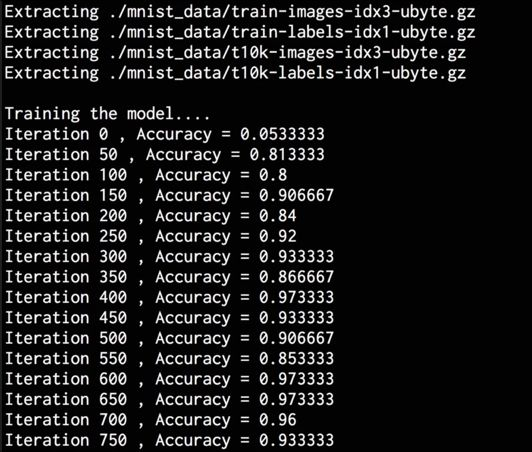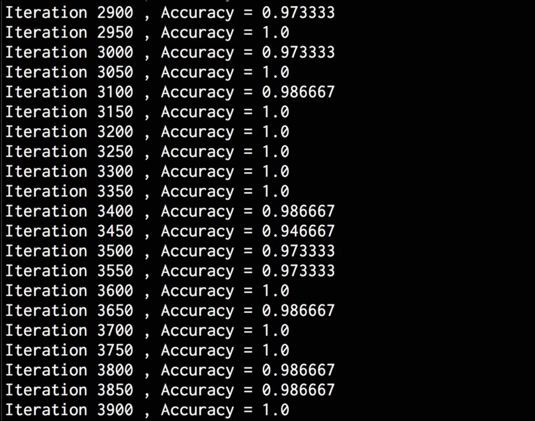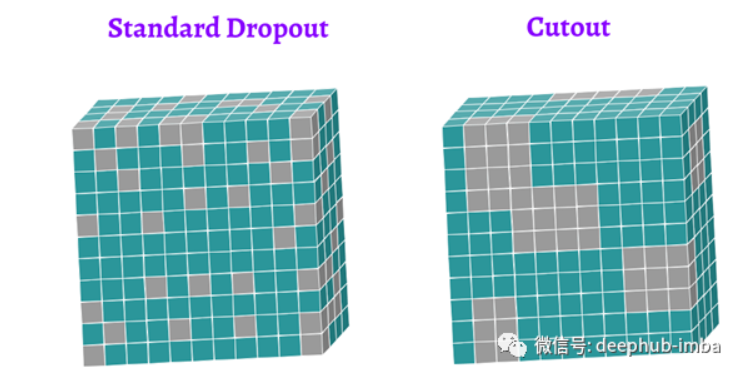DNN、CNN和RNN的12种主要dropout方法的数学和视觉解释（三）
DNN、CNN和RNN的12种主要dropout方法的数学和视觉解释（三）
65 0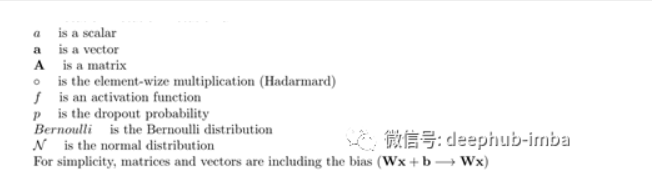DNN、CNN和RNN的12种主要dropout方法的数学和视觉解释（一）
DNN、CNN和RNN的12种主要dropout方法的数学和视觉解释（一）
26 0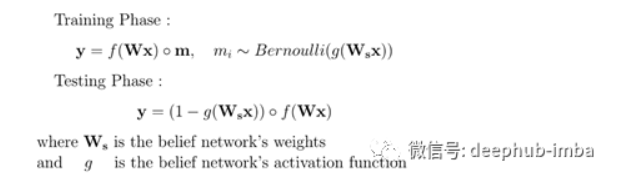DNN、CNN和RNN的12种主要dropout方法的数学和视觉解释（二）
DNN、CNN和RNN的12种主要dropout方法的数学和视觉解释（二）
61 0python深度学习之卷积神经网络(CNN)的使用，包括卷积与池化（附源码，超详细）
python深度学习之卷积神经网络(CNN)的使用，包括卷积与池化（附源码，超详细）
68 0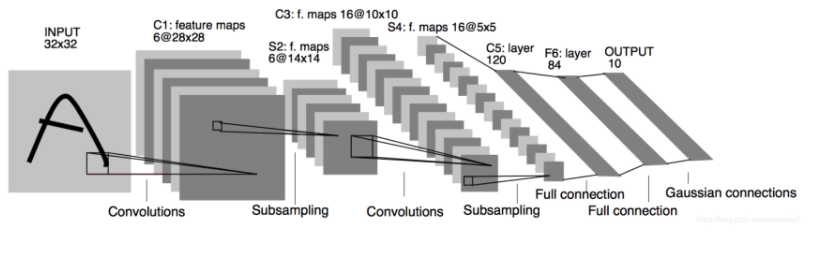91 0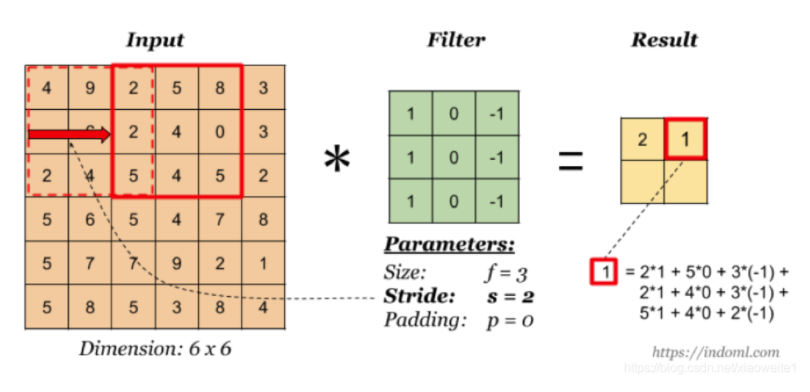73 07053 02816 07512756936265

【算法实战】6. 支持向量机算法5310

【算法实战】4. 朴素贝叶斯算法99643191